summary of site-wide JavaScript functionalityUnited States-English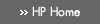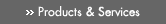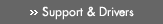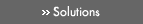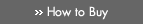» Contact HPSearch:More optionsManualTechnical documentation - EnglishAll of HP USHP-UX Reference > P# pow(3M)

HP-UX 11i Version 3: February 2007»

## Technical documentation» Feedback## » Index## NAME

pow(), powf(), powl(), poww(), powq(), pown(), pownf(), pownl(), pownw(), pownq(), powlln(), powllnf(), powllnl(), powllnw(), powllnq() — power functions

## SYNOPSIS

#include <math.h>

double pow(double x, double y);

float powf(float x, float y);

### HP Integrity Server Only

long double powl(long double x, long double y);

extended poww(extended x, extended y);

double pown(double x, int y);

float pownf(float x, int y);

long double pownl(long double x, int y);

extended pownw(extended x, int y);

double powlln(double x, long long y);

float powllnf(float x, long long y);

long double powllnl(long double x, long long y);

extended powllnw(extended x, long long y);

## DESCRIPTION

The pow() function returns xy.

powf() is a float version of pow(); it takes float arguments and returns a float result.

### Integrity Server Only

powl() is a long double version of pow(); it takes long double arguments and returns a long double result.

poww() is an extended version of pow(); it takes extended arguments and returns an extended result.

powq() is equivalent to powl() on HP-UX systems.

The pown() function returns xy.

pownf() is a float version of pown(); it takes a float first argument and returns a float result.

pownl() is a long double version of pown(); it takes a long double first argument and returns a long double result.

pownw() is an extended version of pown(); it takes an extended first argument and returns an extended result.

pownq() is equivalent to pownl() on HP-UX systems.

The powlln() function returns xy.

powllnf() is a float version of powlln(); it takes a float first argument and returns a float result.

powllnl() is a long double version of powlln(); it takes a long double first argument and returns a long double result.

powllnw() is an extended version of powlln(); it takes an extended first argument and returns an extended result.

powllnq() is equivalent to powllnl() on HP-UX systems.

## USAGE

To use these functions, compile either with the default -Ae option or with the -Aa and -D_HPUX_SOURCE options.

To use (for Integrity servers) poww(), powq(), pownw(), pownq(), powllnw(), or powllnq(), compile also with the -fpwidetypes option.

To use any of these functions, make sure your program includes <math.h>, and link in the math library by specifying -lm on the compiler or linker command line.

For more information, see the HP-UX floating-point guide for HP Integrity servers at the following site: http://www.hp.com/go/fp.

### PA-RISC Only

Millicode versions of the pow() function are available. Millicode versions of math library functions are usually faster than their counterparts in the standard library. To use these versions, compile your program with the +Olibcalls or the +Oaggressive optimization option.

For special cases, the millicode versions return the same values as their standard library counterparts, but do not set errno. (See the RETURN VALUE section.)

## RETURN VALUE

### Integrity System

For Integrity servers, the pow() function follows the C99 specification for special cases:

pow(±0,y) returns ±Inf and raises the divide-by-zero floating-point exception for y an odd integer < 0.

pow(±0,y) returns +Inf and raises the divide-by-zero floating-point exception for y<0 and not an odd integer.

pow(±0,y) returns ±0 for y an odd integer > 0.

pow(±0,y) returns +0 for y>0 and not an odd integer.

pow(-1,±Inf) returns 1.

pow(+1,x) returns 1 for any x, even a NaN.

pow(x,±0) returns 1 for any x, even a NaN.

pow(x,y) returns a NaN and raises the invalid floating-point exception for finite x<0 and finite non-integer y.

pow(x,-Inf) returns +Inf for |x|<1.

pow(x,-Inf) returns +0 for |x|>1.

pow(x,+Inf) returns +0 for |x|<1.

pow(x,+Inf) returns +Inf for |x|>1.

pow(-Inf,y) returns -0 for y an odd integer < 0.

pow(-Inf,y) returns +0 for y<0 and not an odd integer.

pow(-Inf,y) returns -Inf for y an odd integer > 0.

pow(-Inf,y) returns +Inf for y>0 and not an odd integer.

pow(+Inf,y) returns +0 for y<0.

pow(+Inf,y) returns +inf for y>0.

Otherwise, if either argument is a NaN, then pow() returns a NaN.

pow() returns infinity in lieu of a value whose magnitude is too large, and raises the overflow and inexact exceptions.

pow() raises the underflow and inexact exceptions whenever a result is tiny (essentially denormal or zero) and thereby suffers loss of accuracy, and may raise those exceptions if the result is merely tiny.

pow() raises the inexact exception whenever a rounded result does not equal the mathematical result.

Return values and errno settings for pow() that match the PA-RISC behavior specified below can be obtained on Integrity servers by compiling with the +Olibmerrno option.

The pown() and powlln() functions follow the specification for the pow() function in the applicable special and error cases.

### PA-RISC

If x and y are both zero, pow() returns 1.0.

If x or y is NaN, pow() returns NaN.

If x is zero and y is a negative finite number, pow() returns -HUGE_VAL (equal to -INFINITY).

If x is finite and less than zero and y is finite and not an integer, pow() returns NaN.

If the correct value would overflow, pow() returns ±HUGE_VAL (equal to +/-INFINITY).

## ERRORS

If x is zero and y is less than zero, pow() sets errno to [EDOM].

If x is finite and less than zero and y is finite and not an integer, pow() sets errno to [EDOM].

If the correct value would overflow, pow() sets errno to [ERANGE].

HP-UX libm functions on Integrity servers do not set errno by default. For errno setting, compile with the +Olibmerrno option.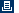Printable versionPrivacy statement Using this site means you accept its terms Feedback to webmaster© 1983-2007 Hewlett-Packard Development Company, L.P.